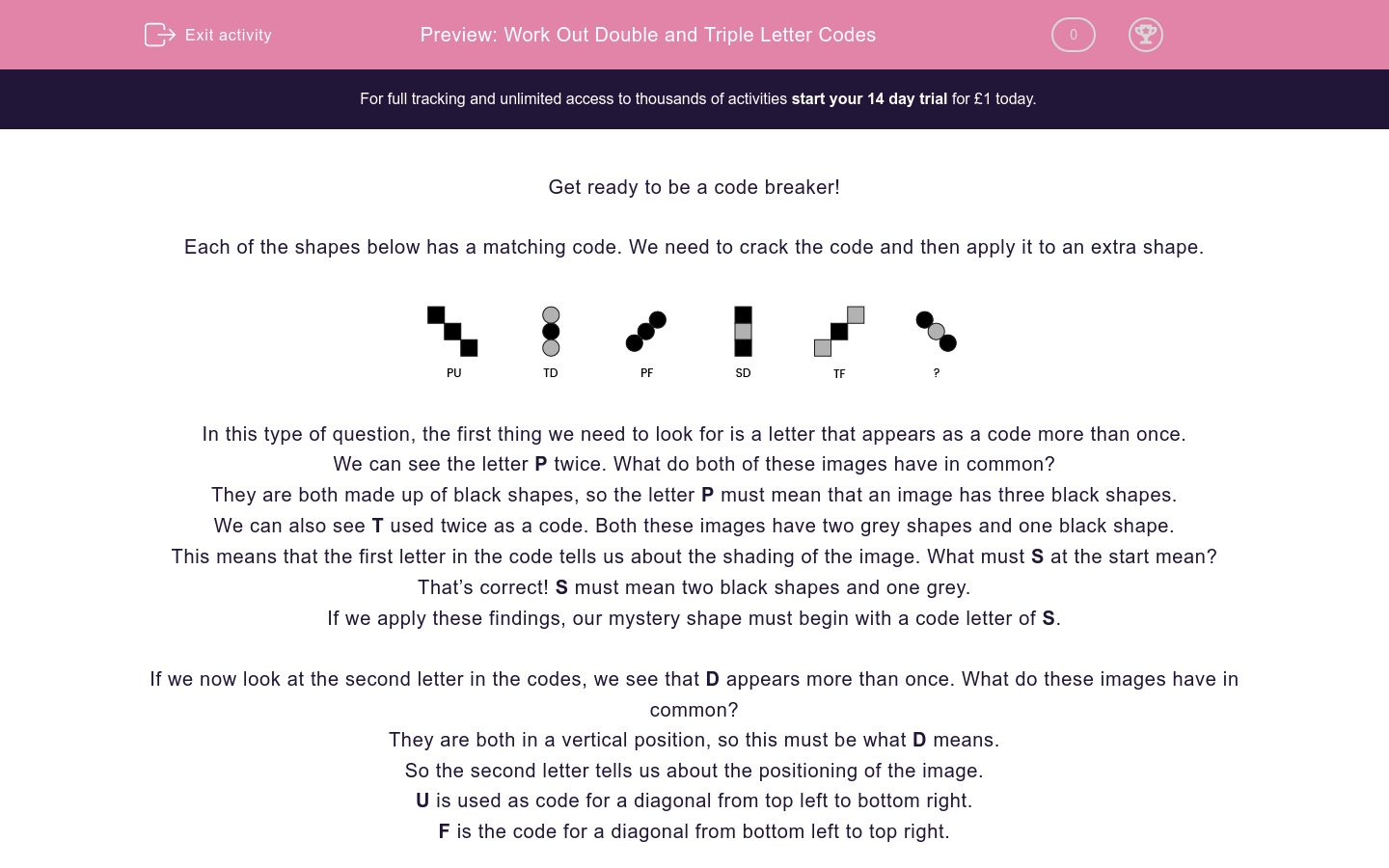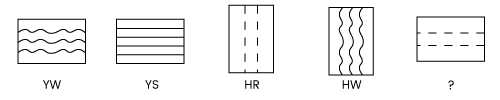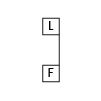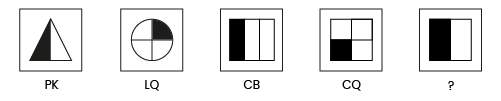# Find Double and Triple Letter Codes

In this worksheet, students will identify double and triple letter codes by identifying common features among images.Key stage:  KS 2

Curriculum topic:   Spatial and Non-Verbal Reasoning

Curriculum subtopic:   Find the Missing Code

Difficulty level:### QUESTION 1 of 10

Get ready to be a code breaker!

Each of the shapes below has a matching code. We need to crack the code and then apply it to an extra shape.In this type of question, the first thing we need to look for is a letter that appears as a code more than once.

We can see the letter P twice. What do both of these images have in common?

They are both made up of black shapes, so the letter P must mean that an image has three black shapes.

We can also see T used twice as a code. Both these images have two grey shapes and one black shape.

This means that the first letter in the code tells us about the shading of the image. What must S at the start mean?

That’s correct! S must mean two black shapes and one grey.

If we apply these findings, our mystery shape must begin with a code letter of S.

If we now look at the second letter in the codes, we see that D appears more than once. What do these images have in common?

They are both in a vertical position, so this must be what D means.

So the second letter tells us about the positioning of the image.

U is used as code for a diagonal from top left to bottom right.

F is the code for a diagonal from bottom left to top right.

Therefore, the code for our final shape is SU.

S for two black shapes and one grey shape, and U for a diagonal from top left to bottom right.

Let’s try another one with a three-letter code this time:

Which code should the final shape have?We can see the letter J appears twice, what do these two images have in common?

They both have an unshaded shape inside the main shape. This means that H is used for a shaded shape on the inside and J is used for an unshaded shape on the inside. If we apply this logic, then our mystery image must have an H in the middle of its code.

What do the two images with a G in their code have in common?

They both have circles inside, so G means a circle inside and S means a square inside. If we apply this logic, then our mystery image should have an S at the end of its code.

Finally, what could the first letter of the code stand for as they are all different?

The only other feature that changes between the images is the type of shape used as the outer shape.

The letter Z must be used for an oval, which is the same as our mystery image.

If we bring this all together, our mystery image should have the code ZHS.

Z - oval on the outside

H - shaded shape on the inside

S - square on the inside

It’s now your turn to crack some codes!

Good luck detective.

Welcome code breaker, we're pleased to see you!

Have a look at the shapes below.

Can you match the code to the correct description?## Column B

U
Thin Shape
D
Vertical Shape
F
Thick Blank Shape
B
Diagonal Shape
G
T
Horizontal Shape

OK, one down - are you ready for the next?

Have a look at the shapes below, code breaker.

Can you match the code to the correct description?## Column B

W
Spotty Shape
R
Rectangle
Z
Circle
S
Triangle
L
D
Striped Shape

Here's another puzzle for you to have a go at, code breaker.

Can you work out the code for the mystery symbol?Look at the following shapes.

Use their codes to help you work out the code for the mystery shape.Let's keep going with this, code breaker, you're on a roll!

Can you work out the code for the mystery symbol?Look at the shapes below.

Use their codes to help you work out the code for the mystery shape.Here's another challenging one for you, code cracker.

Look at the shapes below.

Use their codes to help you work out the code for the mystery shape.Have a look at the shapes below, code breaker.

Can you match the code to the correct description?## Column B

A
Triangles Either End Of The Line
D
Dashed Line
E
Circles Either End Of The Line
H
Full Line
L
Squares Either End Of The Line
M
Wiggly Line

Are you ready for the next challenge, code breaker?

Look at the shapes below.

Use their codes to help you work out the code for the mystery shape.Last question coming up!

Have a look at the shapes below, code breaker.

Can you match the code to the correct description?## Column B

B
Inside Shape Is Blank
G
Inside Shape Is A Square
T
Outside Shape Is A Circle
S
W
Outside Shape Is A Triangle
F
Inside Shape Is A Triangle
K
Outside Shape Is A Square
• Question 1

Welcome code breaker, we're pleased to see you!

Have a look at the shapes below.

Can you match the code to the correct description?## Column B

U
Vertical Shape
D
Diagonal Shape
F
Horizontal Shape
B
Thick Blank Shape
G
T
Thin Shape
EDDIE SAYS
OK code breaker, how did you do? Don't be put off by the fact that the letters of the code are written at the top and bottom of the shapes, rather than underneath the images. You can still crack the codes the same way! What do you notice about the two images with U at the top of their codes? They are both in an upright position. We call this vertical. This means that the top letter of the code tells us about the positioning of the shape. U = vertical D = diagonal F = horizontal Now, let's have a look at the bottom letters of the codes. The images with B at the bottom of their codes are thick blank rectangles. This means that the bottom letter of the code tells us about the shape itself. B = thick blank rectangle G = thick shaded rectangle T = thin line Remember to always look for a letter that appears more than once! Did you follow this? If you didn't get it right first time, don't worry - these things take practice!
• Question 2

OK, one down - are you ready for the next?

Have a look at the shapes below, code breaker.

Can you match the code to the correct description?## Column B

W
Circle
R
Rectangle
Z
Triangle
S
Spotty Shape
L
Striped Shape
D
EDDIE SAYS
Did you crack the code here? All of the images with a W at the beginning are circles. This means that the first letter of the code tells us the shape type. W = circle R = rectangle Z = triangle Now, let's look at the second letter of the codes. Those with L at the end are striped shapes. This means that the second letter of the code tells us about the pattern inside the shape. L = striped S = dotty D = shaded Code cracked! Keep up the hard work, code breaker!
• Question 3

Here's another puzzle for you to have a go at, code breaker.

Can you work out the code for the mystery symbol?YR
EDDIE SAYS
Did you manage to crack the code? Remember to always start by looking for the pattern between shapes with the same code. The rectangles with Y at the front of their codes are both horizontal in position. This means that the first letter of the code tells us about the position of the shape. Y = horizontal H = vertical Now, let's look at the second letter of the code. The rectangles with W at the end of their codes have wiggly lines inside them. This means that the second letter tells us the pattern inside the shape. W = wiggly lines S = straight lines R = dashed lines The mystery shape is in a horizontal position (Y) and has a dashed line (R), so the code that we need here is YR. Keep using those detective skills!
• Question 4

Look at the following shapes.

Use their codes to help you work out the code for the mystery shape.EDDIE SAYS
Did you manage to decode our shapes here? The code was a slightly different layout but it works just the same way as the others, so do not panic! First, we need to look for that common letter among the top codes. We can see T twice. Both of these images have two segments shaded. T = two segments shaded L = one segment shaded Now, let's look at the bottom codes. There are two F's and these are both split into quarters. This means that the second letter tells us how many segments there are in total. F = four segments E = eight segments Q = two segments The mystery shape has one segment shaded (L) and eight segments in total (E), so the code is LE and can be found in the second option.
• Question 5

Let's keep going with this, code breaker, you're on a roll!

Can you work out the code for the mystery symbol?EDDIE SAYS
Did you crack the code here? First, we need to look for that common letter among the top codes. We can see C twice. What do these shapes have in common? They both have three small lines coming out of them. This means that the first letter tells us how many small lines there are. C = three small lines G = four small lines J = five small lines Now, let's look at the bottom codes. We can see W twice and both of these lines are diagonal. The bottom code tells us about the position of the line. W = diagonal F= horizontal U = vertical The mystery shape has three small lines (C) and is vertical (U), so its code is CU, which is the third option. We'll CU on the next question!
• Question 6

Look at the shapes below.

Use their codes to help you work out the code for the mystery shape.KN
EDDIE SAYS
What a LOVEly question! What do you notice about the love hearts with the K at the beginning of their codes? They are made up of three layers. This means that the first letter of the code tells us how many layers the shape has. K = three layers F = four layers P = two layers Now, let's look at the bottom codes. We can see that the letter D appears twice and both of these images have black shaded hearts in the middle. The second letter of the code tells us about the shading of the heart in the middle. D = heavily shaded - black L = lightly shaded - grey N = no shading The mystery shape has three layers (K) and a blank heart in the middle (N), so its code is KN. Excellent code cracking!
• Question 7

Here's another challenging one for you, code cracker.

Look at the shapes below.

Use their codes to help you work out the code for the mystery shape.CK
EDDIE SAYS
How did you do with this one? What do the two shapes with C at the beginning have in common? They are both rectangles. The first code stands for the type of shape. C = rectangle L = circle P = triangle Now, let's look at the second letter of the codes. Both of the shapes with Q at the end of their codes have one quarter shaded. The second letter of the code tells us what fraction of the shape is shaded. Q = one quarter K = one half B = one third The mystery shape is a rectangle (C) and has one half shaded (K), so the code is CK. Keep up the great work, code breaker - you've got this!
• Question 8

Have a look at the shapes below, code breaker.

Can you match the code to the correct description?## Column B

A
Circles Either End Of The Line
D
Squares Either End Of The Line
E
Triangles Either End Of The Line
H
Wiggly Line
L
Dashed Line
M
Full Line
EDDIE SAYS
How many matches did you find? Let's look at the letter A, as it comes up twice. Both of these images have circles either end of the line. This means that the first letter of the code stands for which shape is at the ends of the line. A = circles D = squares E = triangles Now, let's look at the second letters. We can see L twice and both of these have dashed lines. The second letter of the code tells us about the type of line. L = dashed line H = squiggly line M = full line Always look for a common letter and work from there.
• Question 9

Are you ready for the next challenge, code breaker?

Look at the shapes below.

Use their codes to help you work out the code for the mystery shape.UCR
EDDIE SAYS
Wow, we had three letters to decode here! How did you manage? First, let's look at the front of the code. The two images with U at the front of their codes have an arrow pointing upwards. The first letter tells us which way the arrow is pointing. U = arrow pointing up D = arrow pointing down Now, let's look at the middle letters. Both of the images with W in the middle have a blank shape. The middle letter tells us about the shading of the small shape. W = blank shape C = shaded shape Finally, let's look at the last letter. Two of the last letters are S and both of these images have a square. The last letter tells us which type of shape is inside. S = square T = triangle R = circle The mystery shape has an arrow pointing up (U), a shaded shape (C) and a circle (R), so the code we need is UCR. Did you keep up? These ones are tricky but the more you do, the easier they will become.
• Question 10

Last question coming up!

Have a look at the shapes below, code breaker.

Can you match the code to the correct description?## Column B

B
Outside Shape Is A Triangle
G
Outside Shape Is A Square
T
Outside Shape Is A Circle
S
W
Inside Shape Is Blank
F
Inside Shape Is A Square
K
Inside Shape Is A Triangle
EDDIE SAYS
Lots of codes to crack here! Let's start with the first letters. The images with G at the start have squares on the outside. The first letter tells us the outside shape. G = square on the outside B = triangle on the outside T = circle on the outside The middle letter tells us if the inside shape is shaded or not. S = shaded W = blank The last letter of the code is about the type of shape on the inside. F = square inside K = triangle inside. Codes cracked! Great job, detective - that is another activity solved!
---- OR ----

Sign up for a £1 trial so you can track and measure your child's progress on this activity.

### What is EdPlace?

We're your National Curriculum aligned online education content provider helping each child succeed in English, maths and science from year 1 to GCSE. With an EdPlace account you’ll be able to track and measure progress, helping each child achieve their best. We build confidence and attainment by personalising each child’s learning at a level that suits them.

Get started